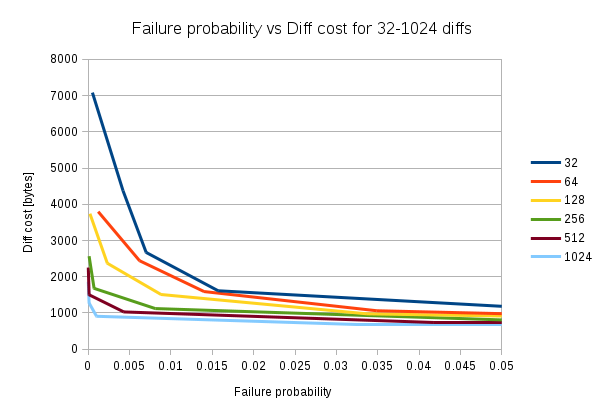## IBLT, Part III: Failure probability and scaling

<< Part II | This is Part III of a multi-part series on Bitcoin block propagation with IBLT. | Part IV >>

I want to get idea on how failure probability, the probaility that decoding of the IBLT fails, depends on cellCount. So the question is

How fast does failure probability drop when the IBLT size increase?

To answer this, I encoded a certain amount of differences (equal amount of absent and extra transactions), where the transactions were selected randomly from a 1000 block span on the blockchain. The IBLT is then decoded to see if it succeeds or fails. The process is repeated 10000-100000 times with new random transactions in each sample. I did the same test for seven different diff cell Counts for 6 different (32-1024) diff counts.

for i = 0..5 // For each diff count
diffCount = 32 \* 2^i
cellCount = findGoodStartValue()
for j=0..6 // For each cell count
cellCount = calculateStep() // Some arbitrary step
samples = j > 3 ? 100000 : 10000
for k=1..samples // For each sample
extra = select diffCount/2 random transaction
absent = select diffCount/2 random transactions not in extra
runSample(cellCount, extra, absent)

Enough said, here are the graphs: